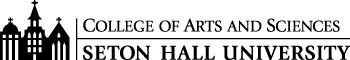# Outcomes - B.S., MathematicsSeton Hall University embraces the principle that effective and meaningful assessment is an integral part of the educational process. This principle is at the heart of our commitment to meet our responsibilities to our students, professions, and the communities that we serve.

1. Knowledge of Problem Solving. Students know, understand and apply the process of mathematical problem solving.
2. Knowledge of Reasoning and Proof, Students reason, construct, and evaluate mathematical arguments and develop as appreciation for mathematical rigor and inquiry.
3. Knowledge of Mathematical Communication. Students communicate their mathematical thinking orally and in writing to peers, faculty and others.
4. Knowledge of Mathematical Connections. Students recognize, use, and make connections between and among mathematical ideas and in contexts outside mathematics to build mathematical understanding.
5. Knowledge of Technology. Students can employ technology appropriately for doing mathematics.
6. Knowledge of Number and Operations. Students demonstrate computational proficiency, including a conceptual understanding of numbers, ways of representing number, relationships among number and number systems, and the meaning of operations.
7. Knowledge of Algebra. Students demonstrate a computational and conceptual understanding of the axiomatic structure of vector spaces, groups, rings and fields.
8. Knowledge of Geometries. Students use spatial visualization and geometric modeling to explore and analyze geometric shapes, structures, and their properties.
9.  Knowledge of Analysis.  Students demonstrate a computational and conceptual understanding of limit, continuity, differentiation, and integration and gain a thorough background in techniques and application of analysis and the mathematical idea of the infinite.
10. Knowledge of Discrete Mathematics. Students apply the fundamental ideas of discrete mathematics in the formulation and solution of problems.
11. Knowledge of Data Analysis, Statistics and Probability. Students demonstrate an understanding of concepts and practices related to data analysis, statistics, and probability.